#There are 366 different Starters of The Day, many to choose from. You will find below some starters on the topic of Graphs. A lesson starter does not have to be on the same topic as the main part of the lesson or the topic of the previous lesson. It is often very useful to revise or explore other concepts by using a starter based on a totally different area of Mathematics.

Main Page

### Graphs Starters: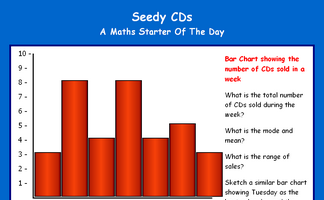Interpret data given in the form of a bar chart then create a new bar chart showing the given statistics.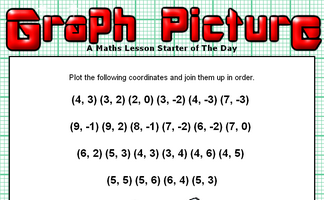Plot the given coordinates then join them up to form a picture.An exercise in estimating what the sectors of a pie chart represent.

## Exercises#### A Show Of Hands

Produce a number of graphs and charts from a quick show of hands.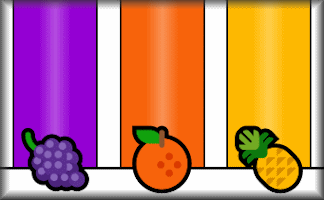#### Bar Charts

Practise constructing and interpreting bar charts for everyday situations.#### Cartoon Scatter Graph

Place the cartoon characters on the scatter graph according to their height and age.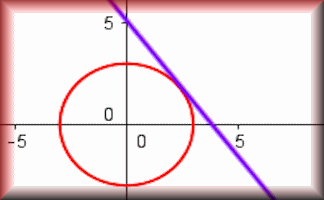#### Circle Equations

Recognise and use the equation of a circle with centre at the origin and the equation of a tangent to a circle.#### Comparing Graphs

Would you recognise a misleading graph if you saw one? Try this comparative judgement exercise to rate statistical graphs.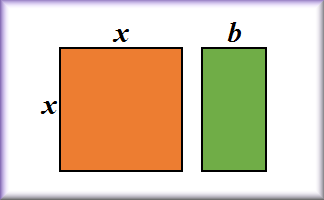#### Completing the Square

Practise this technique for solving quadratic equations and analysing graphs.#### Coordinate Geometry Table

Fill in the empty cells of this table with information about lines, gradients and coordinates.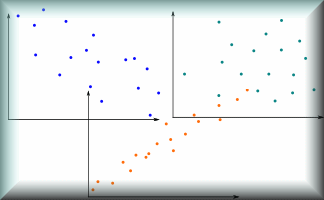#### Correlation

Arrange the given statements in groups to show the type of correlation they have.#### Deconstructing Graphs

Fill in the tables of values from the information that can be read from the given graphs.#### Differentiation

Practise the technique of differentiating polynomials with this self marking exercise.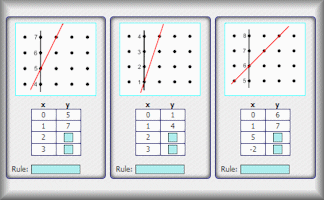#### Don's Graph Snaps

Complete the tables and find the equations of the graphs that can be seen in the snaps.#### Equation of a Line Through Points

Match the equations of the straight line graphs to the clues about gradients and points.#### Estimating Correlation

Practise the skill of estimating the correlation of data on a scatter graph in this self marking exercise.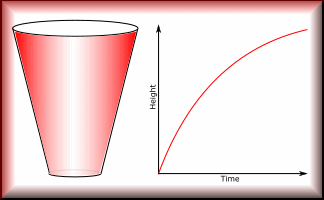#### Fill Graph Pairs

If the container is gradually filled with a steady flow of water which height-time graph would be produced?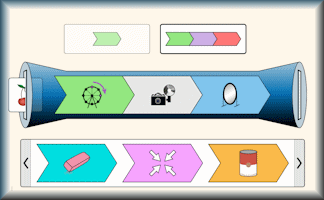#### Function Builder

An interactive function machine for patterns, numbers and equations.Practise the skill of finding the gradients of straight lines by counting squares and dividing rise by run.#### Graph Equation Pairs

Match the equation with its graph. Includes quadratics, cubics, reciprocals, exponential and the sine function.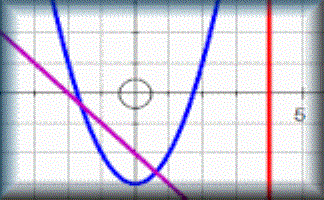#### Graph Match

Match the equations with the images of the corresponding graphs. A drag-and-drop activity.#### Graph Paper

Flexible graph paper which can be printed or projected onto a white board as an effective visual aid.#### Graph Patterns

Find the equations which will produce the given patterns of graphs.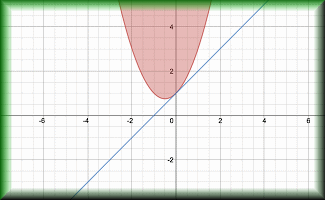#### Graph Plotter

An online tool to draw, display and investigate graphs of many different kinds.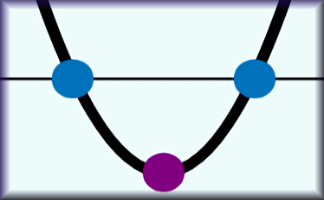Describe how changing the coefficients of a quadratic function changes the graph of the function#### Graphs of Vertical and Horizontal Lines

Questions about the equations of straight line graphs that are parallel to the axes.#### Helicopter View

An animated introduction to distance-time graphs. Arial photographs of vehicles moving along a road placed side to side form a graph.#### Histograms

Practise drawing and reading information from histograms displaying grouped data#### Human Graphs

Students should be encouraged to stand up and make the shapes of the graphs with their arms.#### Human Scatter Graphs

Pupils move to positions in the room according to their data relative to the walls as axes.#### Hurdles Race

An animated distance time graph to be viewed while a student interprets the graph and comments on the race that produced the graph.#### Integration

Exercises on indefinite and definite integration of basic algebraic and trigonometric functions.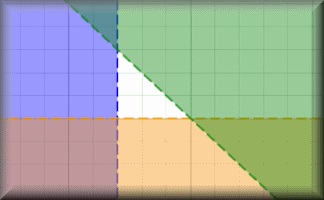#### Linear Programming

A selection of linear programming questions with an interactive graph plotting tool.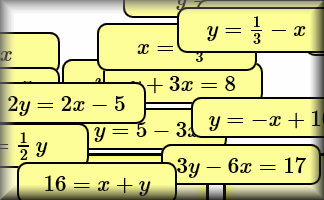#### Parallel Graphs

Collect together in groups the equations of the graphs that are parallel to each other.#### Perpendicular Pairs

Find the pairs of equations that will produce perpendicular graphs.#### Pie Chart Creator

A quick and convenient tool for rapidly creating simple pie charts.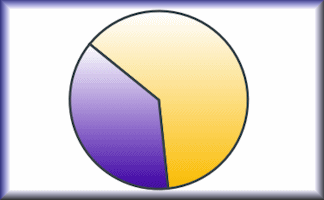#### Pie Charts

Develop the skills to construct and interpret pie charts in this self-marking set of exercises.#### Plotting Graphs

Complete a table of values then plot the corresponding points to create a graph.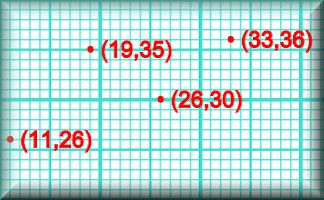#### Plotting Scatter Graphs

Plot scatter graphs from data representing a number of different everyday situations.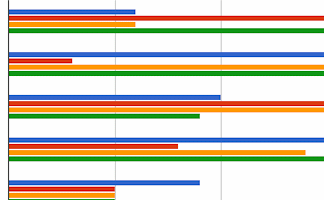Answer real-life problems from different types of graphs and charts including piece-wise linear graphs.#### Straight Line Graph Equation

An online exercise about the equation y=mx+c and the features of a straight line graph.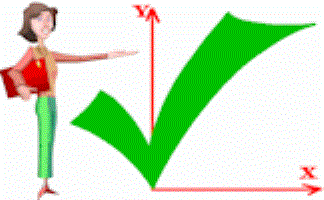#### Straight Line Graphs

10 straight line graph challenges for use with computer graph plotting software or a graphical display calculator.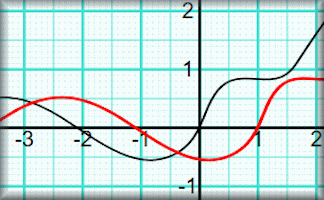#### Transformations of Functions

A visual aid showing how various transformations affect the graph of a function.#### Travel Graphs

Test your understanding of distance-time and speed-time graphs with this self-marking exercise.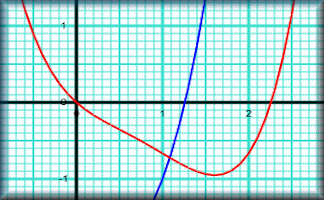#### Using Graphs

Use the graphs provided and create your own to solve both simultaneous and quadratic equations.#### Yes No Questions

A game to determine the mathematical item by asking questions that can only be answered yes or no.

### Search

The activity you are looking for may have been classified in a different way from the way you were expecting. You can search the whole of Transum Maths by using the box below.

Have today's Starter of the Day as your default homepage. Copy the URL below then select
Tools > Internet Options (Internet Explorer) then paste the URL into the homepage field.

Set as your homepage (if you are using Internet Explorer)

Do you have any comments? It is always useful to receive feedback and helps make this free resource even more useful for those learning Mathematics anywhere in the world. Click here to enter your comments.For All: